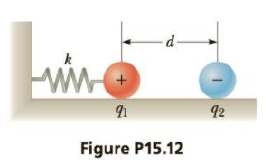Chapter 15, Problem 12P

Chapter
Section
Textbook Problem

A positive charge q1 = 2.70 μC on a frictionless horizontal surface is attached to a spring of force constant k as in Figure P15.12. When a charge of q2 = −8.60 μC is placed 9.50 cm away from the positive charge, the spring stretches by 5.00 mm, reducing the distance between charges to d = 9.00 cm. Find the value of k.To determine
The value of spring constant.

Explanation

Given info: The two charges q1=2.70μC and q2=8.60μC The distance of separation (d) between the charges is 9.00 cm. The displacement (x) of the spring is 5.00 mm.

Formula to calculate the spring force is,

F=kx (I)

• k is the spring constant.

From Coulomb’s Law,

F=ke|q1||q2|d2 (II)

• ke is the Coulomb constant.
• q1 and q2 are the charges.
• d is the distance of separation.

The spring force equals the Coulomb force at equilibrium.

From Equations (I) and (II)

kx=ke|q1||q2|d2

On Re-arranging,

k=ke|q1||q2|xd2

Substitute 8.99×109N.m2/C2 for ke , 2.70μC for q1 , 8

Still sussing out bartleby?

Check out a sample textbook solution.

See a sample solution

The Solution to Your Study Problems

Bartleby provides explanations to thousands of textbook problems written by our experts, many with advanced degrees!

Get Started